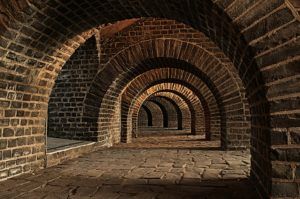## Putting It Together: Conic Sections

At the beginning of this module, you were presented with the challenge of creating a whispering gallery. Now that you know about conics, you can do just this. It turns out that a whispering gallery forms under an elliptical arch.You can create the gallery by placing the whispering dishes at the foci of the ellipse. Let’s place the center of the arch above the origin. The equation for an ellipse centered at the origin is

${\Large\frac{x^2}{a^2}+\frac{y^2}{b^2}}=1$

The width of the arch is 100 feet, so $a=50$. The height of the arch is 40 feet, so $b=40$. Now you can rewrite the ellipse equation.

${\Large\frac{x^2}{2500}}+{\Large\frac{y^2}{1600}}=1$

To find the distance, $c$, from the origin to the foci of the ellipse, use

$\begin{gathered}c^2=a^2-b^2 \\ c^2=50^2-40^2 \\ c^2=900 \\ c=30 \end{gathered}$

So you need to place the whispering dishes 30 feet from the center of the room.

What if the height of the arch were lowered to 30 feet? Could you still make it a whispering gallery?

If this happens, the width of the arch is still 100 feet, so $a=50$. But the height of the arch is 30 feet, so $b=30$.  Now you can rewrite the ellipse equation.

${\Large\frac{x^2}{2500}}+{\Large\frac{y^2}{900}}=1$

To find the distance, c, from the origin to the foci of the ellipse, use

$\begin{gathered} c^2=a^2-b^2 \\ c^2=50^2-30^2 \\ c^2=1600 \\ c=40 \end{gathered}$

You’ll have to move the dishes out another 10 feet on each side so that they are 40 feet from the center. Thanks to your knowledge of conics, you can use the dimensions of the arch to place the dishes where they belong to create a whispering gallery. Now, just be careful not to share any secrets you don’t want anyone to hear.